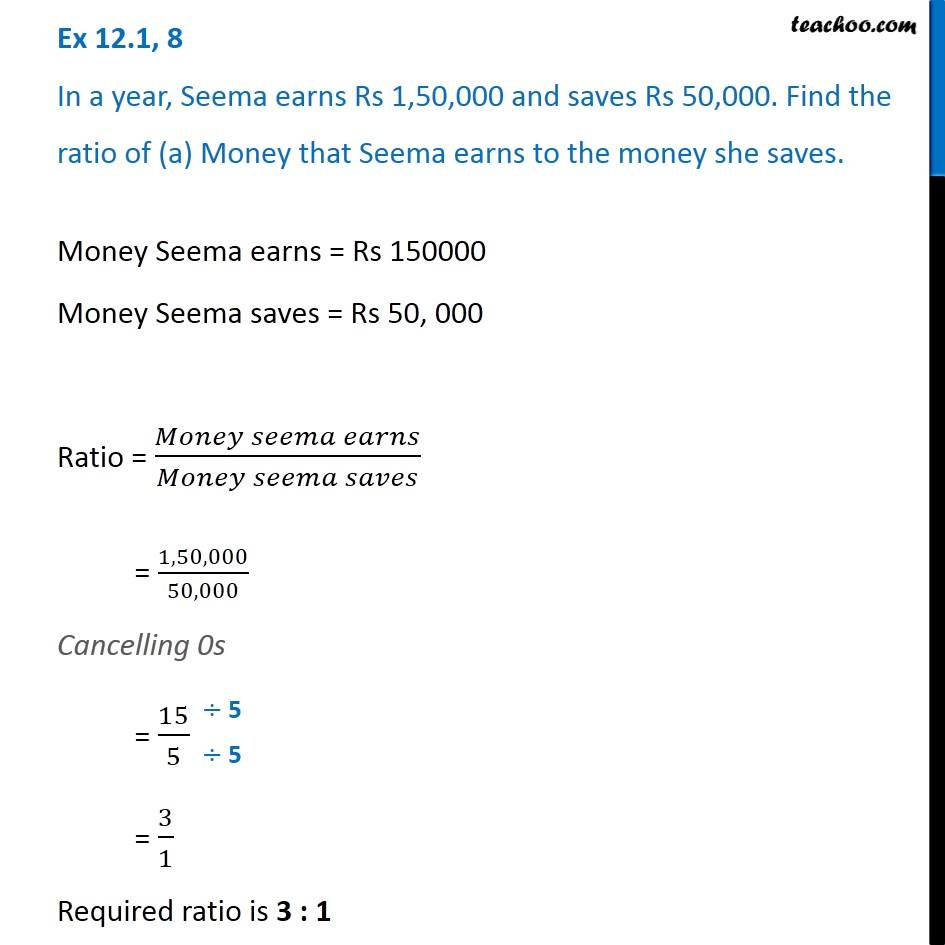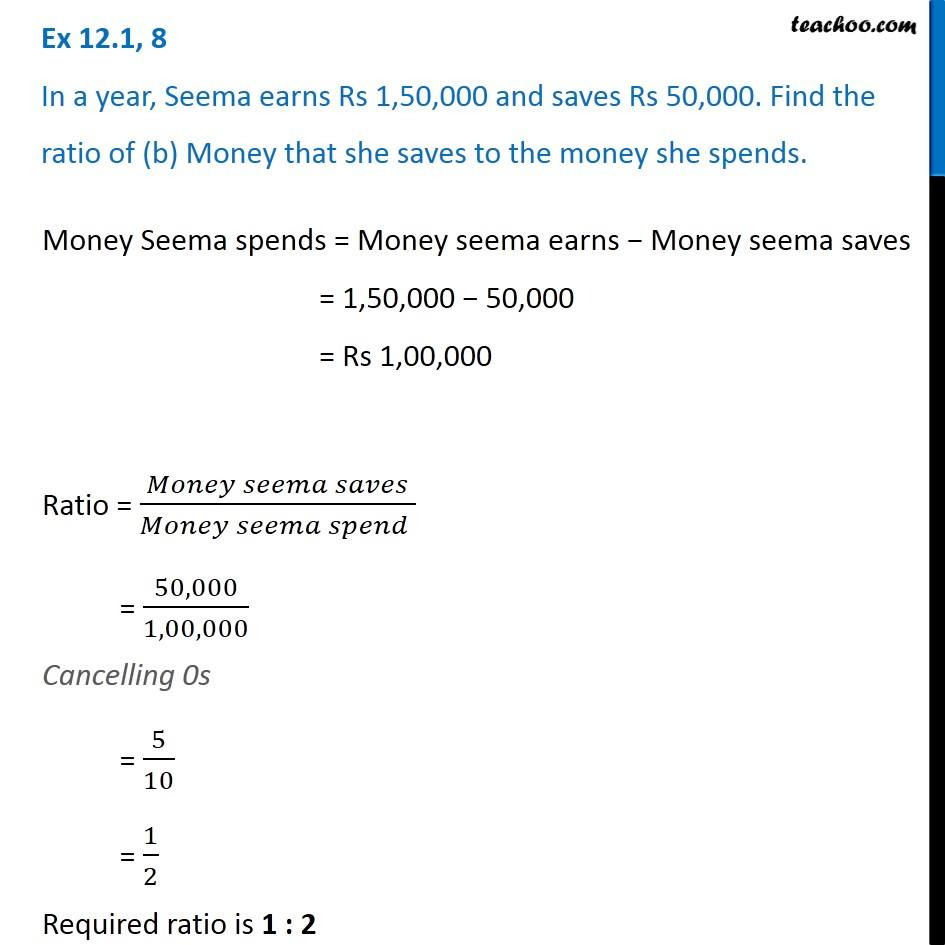1. Chapter 12 Class 6 Ratio And Proportion
2. Serial order wise
3. Ex 12.1

Transcript

Ex 12.1, 8 In a year, Seema earns Rs 1,50,000 and saves Rs 50,000. Find the ratio of (a) Money that Seema earns to the money she saves Money Seema earns = Rs 1,50,000 Money Seema saves = Rs 50,000 Ratio = Money Seema earns / Money Seema saves = 1,50,000 / 50,000 Cancelling 0's = 15/5 = 3/1 Required ratio is 3:1 (b) Money that she saves to the money she spends. Money Seema spends = Money seema earns − Money seema saves = 1,50,000 − 50,000 = Rs 1,00,000 Ratio = (Money Seema Saves) / (Money Seema Spends) = 50,000/1,00,000 Cancelling 0s = 5/10 = 1/2 Required ratio is 1 : 2

Ex 12.1

Chapter 12 Class 6 Ratio And Proportion
Serial order wise